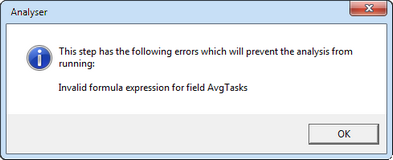﻿ Formula Syntax

# Formula Syntax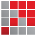When defining a formula expression in the Calculation Editor, whether for an analysis or to calculate results in assessment items, it is vitally important that you use the correct syntax. You must be aware that:

 • A set of general syntax principles applies, eg the use of quotation marks, white space, etc.
 • Each function has its own specific syntax and arguments which must be observed when building your formula.
 • The order in which operations are performed in formulae, especially where they are complex, follows a set of precedence rules.
 • Issues may arise when data that is not numeric is being sorted.
 • Different types of error messages will be encountered when a function cannot be performed.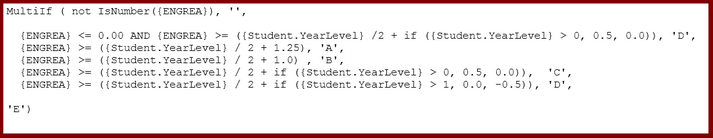General syntax principles

Although each function has its very specific syntax, some general principles apply across the board:

Syntax

Notes

{ }

When you insert a field from the Fields pane into an expression, a set of curly brackets or braces will be inserted around the field, eg:

{Subject.Code}

It is not always necessary to place brackets around field names. However if a field contains embedded spaces or anything other than underscore, hash or alphanumeric characters, you must enclose it in curly brackets, eg if you create a new field which includes spaces that is being used in the expression, eg {New Field}.

( )

Brackets or parentheses enclose all of a function’s instructions, as well as grouping together operations or nested statements.

You must have matching sets of brackets, eg:

 • DCountIf({ItemName}, Left({ItemName},1)=“A”)
 • (Round(({CountBoys}/{CountTotal} * 100), 2))&"%"

" " and  ' '

Sets of either double or single quotation marks must be used around strings, ie non-numeric text, eg:

{SubjectName} = “ENG07”.

When a string needs to be inserted within further quotation marks, the other type of quotes should be used, eg:

CountIf(“ = ‘S’”,{ITEM1},{ITEM2},{ITEM3})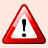Do not enclose field names within quotation marks – use curly brackets.

White space

You may insert spaces and new lines in appropriate places throughout your formulae to make them more readable, although it is not necessary to do so.

As seen below, the MultiIf function is much easier to follow with each of its paired arguments set out on a row each.

However, where a field name is inserted, you must not insert additional embedded spaces within it or its curly brackets.Precedence of operations

Expressions can become quite complex, including a whole series of components, each of which needs to be evaluated. Therefore, it is important that the expression is composed in a way that the evaluation order is correct for the calculation you require.

For example, the result of  1 +  2 * 3  will be different from  (1 + 2) * 3.  The former will evaluate to 7 and the latter to 9. This is because parentheses take precedence over multiplication, which in turn is executed before addition.

The order of precedence of operations when a formula is being calculated, from highest to lowest, is:

 • Expressions within parentheses ( )
 • Concatenate (&)
 • In and Like operators
 • Negation (-x), Logical Not and Bitwise Not
 • Multiply (*) and Divide (/)
 • Add (+) and Subtract (-)
 • Equal (=), Not Equal (<>), Greater than (>), Greater than or equal (>=), Less than (<), Less than or equal (<=)
 • Logical And, Bitwise And
 • Logical Or, Bitwise Or

Where operators have the same precedence, they are executed left to right.It is a good idea to use parentheses to be absolutely certain that an expression is evaluated in the way you want it to be.Function arguments

 • A function name, eg Min, Left, DCountIf.
 • A set of brackets or parentheses, ie ( ).
 • A series of arguments which are required by the function, enclosed within the brackets and separated by commas.

The arguments for each of the available functions will generally be one of the following, although some specialist arguments may exist for some functions. In such cases, you should refer to the explanation of the specific function.

 Arguments Requirement Examples expr or dexpr An expression, which may be an available field or a string or contain operators. Expressions can be numbers or strings. If({Student.Gender} = “M”,“Boy”, “Girl”) If({EnteredValue} = “NA”, “Not Applic”, {EnteredValue}) num or dnum A number is required, or the data stored in the field is a numeric. Left({ItemName}, 2) Round(({PercentBoys}), 3) cond or dcond A condition which may be true or false. DCountIf({Student.Gender}, {Student.Gender} = "M") str or dstr String of characters, which may also be one of the available fields. If a string of characters, it should be enclosed in quotation marks. Left({Student.FamilyName}, 1) Mid(“ABCDEF”,{EnteredValue}, 1)

You should also note the following:

 • Some arguments will be optional, and these are indicated by square brackets, eg [dcond], [, num], etc.
 • All of the functions under the Group branch begin with a D, and their arguments are prefixed by a d, to make them distinguishable from other non-group functions, eg: Dsum(dnum)
 • The Group functions perform calculations across records, eg across all students in a subject or multiple results for a student. In the Analyser window, they are most useful in the Aggregate Operator. As the table of arguments above shows, the arguments preceded by d are, to all intents and purposes, the same as the ones without.Function sorting anomalies

When setting up expressions which are making comparisons, such as when sorting or getting maximum and minimum values, there are a few potential anomalies.

 • In general, data is sorted in the following order of precedence:
 • Blanks
 • Errors
 • Numbers
 • Dates
 • Strings, including alphanumeric characters and spaces
 • Arrays and other objects

 • In the case of strings which are mixed numeric and alphanumeric characters, eg AT1, AT2, 10ENG, etc, a smart sort is applied. For example, a series of assessment item codes from AT1 to AT20 will sort AT1, AT2, AT3 etc, ie the numeric characters are treated as numbers. Likewise, a series of subject codes that combine numbers and alphabetic charcters would sort 8ENG, 9ENG, 10ENG, etc.

This sort method is in comparison to a normal alphanumeric sort which would result in AT1, AT10, AT11, etc.

 • In the case of results which have modifiers after them such as A+, A, A-, the sort order for these is A, A+, A-, B, B+, B-, etc.

In the Analyser, it is best to use the {ResultOrdinal} field when dealing with such values.

For assessment item calculations, use the Ordinal function, in conjunction with the assessment item's List Item Collections field.

For example:

would return the lowest ordinal value any student has received in the subject's TASK1 assessment item.

Keep in mind that the lowest ordinal is usually the highest result, as list marking schemes are generally ordered from highest to lowest.Formula errors

A formula may be set up incorrectly, eg have incorrect syntax, in which case errors will appear. These appear differently if found in assessment items compared to an analysis.Errors in assessment items

When a calculation is being evaluated in an assessment item, it is possible that the Accelerus is unable to evaluate it and return a meaningful result. Instead, an error value is returned. Furthermore, even if the calculation is able to be evaluated, the result may be outside the acceptable values for the assessment item's marking scheme.

Therefore, when Accelerus users are in any of the class, subject or student results windows, these errors are visible on the screen, as shown in this example.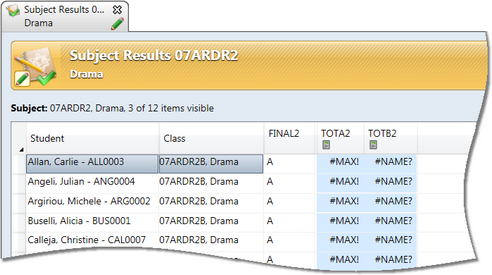When student reports are printed and a particular assessment item's result is incorrect because of a calculation error, the error will not print on the report, but will be substituted by a blank result.

Errors relating to the evaluation of calculations in assessment items that you may encounter include:

 Displayed error Cause #ARRAY! A multi-valued expression has been used where only a single value is expected, eg [1,2,3], in an assessment item calculation. #DIV/0! A division by zero has occurred, eg if using an AvgX function and no results in assessment items that the calculation is based upon are valid. #ELEM! An expression or function that has more than one multi-valued expression with a different number of values in each. For example: [1,2,3] + [1,2] OR DSumIf({Subject.AT1}, {Class.AT1} > 50) The first example is trying to add an array of three elements to another array, which only has two elements. In the second example, we are mixing subject results and class results which would have a differing number of elements. #ERR! A general error outside those listed above has occurred. #MAX! The result calculated is greater than the maximum allowed for the numeric marking scheme. #MIN! The result calculated is less than the minimum allowed for the numeric marking scheme. #NAME! The name of a field or variable referred to in the formula is not recognised. #NUM! A function required a numeric argument, but something other than a number was used. #VALUE! A function argument was outside the expected range of values. Most commonly this is a number that is too big or small. #DATE! The value cannot be translated into a date, where a date marking scheme has been used.Errors in analyses
 When a formula error is encountered in an analysis window, in any of the Formula, Filter or Aggregate operators, which use the Calculation Editor, the particular operator that contains the error will display a red exclamation symbol, as shown below.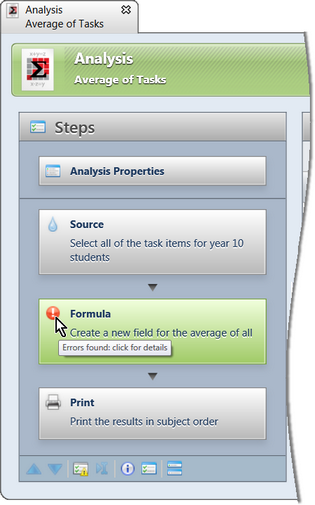If you click the error symbol, details of the error will be displayed.